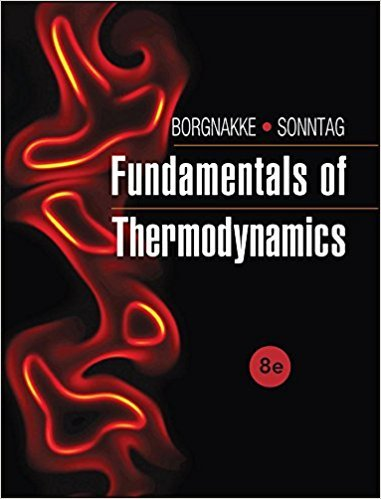×
Get Full Access to Fundamentals Of Thermodynamcs - 8 Edition - Chapter 3 - Problem 183hp
Get Full Access to Fundamentals Of Thermodynamcs - 8 Edition - Chapter 3 - Problem 183hp

×

# A piston/cylinder has 0.5 kg of air at 2000 kPa, 1000 K,ISBN: 9781118131992 58

## Solution for problem 183HP Chapter 3

Fundamentals of Thermodynamcs | 8th Edition

• Textbook Solutions
• 2901 Step-by-step solutions solved by professors and subject experts
• Get 24/7 help from StudySoup virtual teaching assistantsFundamentals of Thermodynamcs | 8th Edition

4 5 1 242 Reviews
20
2
Problem 183HP

Problem 183HP

A piston/cylinder has 0.5 kg of air at 2000 kPa, 1000 K, as shown in Fig. P3.183. The cylinder has stops, so Vmin=0.03m3. The air now cools to 400K by heat transfer to the ambient. Find the final volume and pressure of the air (does it hit the stops?) and the work and heat transfer in the process.

FIGURE P3.183Step-by-Step Solution:

Solution 183HP

Step 1 of 6:

Our aim is to find the final volume, the final pressure, the work and the heat transfer (released) of the air filled cylinder with the piston during the process given.

The mass of air m = 0.5 kg

The initial temperature T1 = 1000 K

The initial pressure P1 = 2000 kPa

The final temperature T2 = 400 K

The gas constant R = 0.287 kJ/kg.K        (table A.5)

The minimum volume at the final stop Vmin = 0.03 m3

Step 2 of 6

Step 3 of 6

##### ISBN: 9781118131992

Unlock Textbook Solution